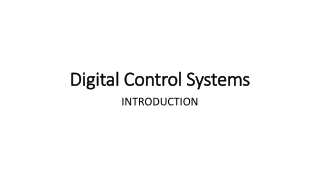DownloadDownload PresentationDigital Control Systems

# Digital Control Systems

Télécharger la présentation## Digital Control Systems

- - - - - - - - - - - - - - - - - - - - - - - - - - - E N D - - - - - - - - - - - - - - - - - - - - - - - - - - -
##### Presentation Transcript

1. Digital Control Systems INTRODUCTION

2. Introduction What is a controlsystem? Objective: To make the system OUTPUT and the desired REFERENCE as close aspossible, i.e., to make the ERROR as small as possible. Key Issues: 1) How to describe the system to be controlled? (Modeling) 2) How to design the controller? (Control)

3. Introduction What is important in a controlsystem? Stability • (Transient) responsespeed • Accuracy • dynamic overshooting and oscillation duration • Steadystateerror • Robustness • errors in models (uncertainties and nonlinearities) • effectsof disturbances • effectsof noises

4. Introduction Modeling of dynamicsystems Model: A representation of a system. Types of Models: • Physicalmodels (prototypes) • Mathematical models (e.g., input-output relationships) Analytical models (using physical laws) Computer(numerical) models Experimental models (using input/output experimental data) Models for physical dynamic systems: Lumped-parametermodels Continuous-parameter models. Example: Spring element (flexibility, inertia, damping)

5. Introduction Signal categories for identifying control system types Continuous-time signal & quantizedsignal Continuous-time signal is defined continuously in the time domain. Figure on theleft shows a continuous-time signal, represented by x(t). Quantized signal is a signal whose amplitudes are discrete and limited. Figure onthe right shows a quantized signal. Analog signal or continuous signal is continuous in time and in amplitude. Thereal word consists of analog signals.

6. Introduction Discrete-time signal & sampled-data signal Discrete time signalsampled data signal Discrete-time signal is defined only at certain time instants. For a discrete-time signal,the amplitude between two consecutive time instants is just not defined. Figure on theleftshows a discrete-time signal, represented by y(kh), or simply y(k), where k is aninteger and h is the time interval. Sampled-data signal is a discrete-time signal resulting by sampling a continuous-timesignal. Figure on the right shows a sampled-data signal deriving from thecontinuous-time signal, shown in the figure at the center, by a sampling process. It isrepresentedbyx∗(t).

7. Introduction Digital signal or binary coded data signal Digitalsignal Digital signal is a sequence of binary numbers. In or out from a microprocessor, asemiconductor memory, or a shift register. In practice, a digital signal, as shown in the figures at the bottom, is derived by twoprocesses: sampling and then quantizing.

8. Introduction Control SystemTypes

9. Introduction Mathematical comparison between analog and digital control systems

10. Introduction Digital Control systems Digitalcontrolsareusedforachieving optimal performance-forexample, in the form of maximumproductivity, maximumprofit, minimum cost, or minimum energyuse.

11. Introduction Digital Control systems Digitalcontrolsareusedforachieving optimal performance-forexample, in the form of maximumproductivity, maximumprofit, minimum cost, or minimum energyuse.

12. Introduction Advantagesof digitalcomputers: • Reducedcost, • Flexibility in response to design changes, • Noiseimmunity • Digital control systems are more suitable for Modern control systems. Disadvantages of digitalcomputers: • From the tracking performance side, the analog control system exhibits good performances than digital control system. • Digitalcontrol system will introduce a delay in the loop.

13. Introduction The loop (forward and feedback) contains both analog and digital signals must provide a means for conversion from one form to other to be used by each subsystem. Analog-to-Digital Converter(ADC) A device that converts analog signal to digital signal is called Analog-to-Digital Converter. Digital Analog Converter(DAC) Adevice that converts digital signals to analog signals is called a Digital Analog Converter.

14. Introduction Digital-To-Analog Conversion From the binary number each bit is properly weighted voltages and are summed together to yield analogue output.

15. Introduction Analog-To-Digital Conversion ADC is not instantaneous and needs two-step process. There is a delay between the analog input voltage and the output digital word. In ADC, the analog signal is first converted to a sampled signal and then converted to a sequence of binary numbers, the digital signal.